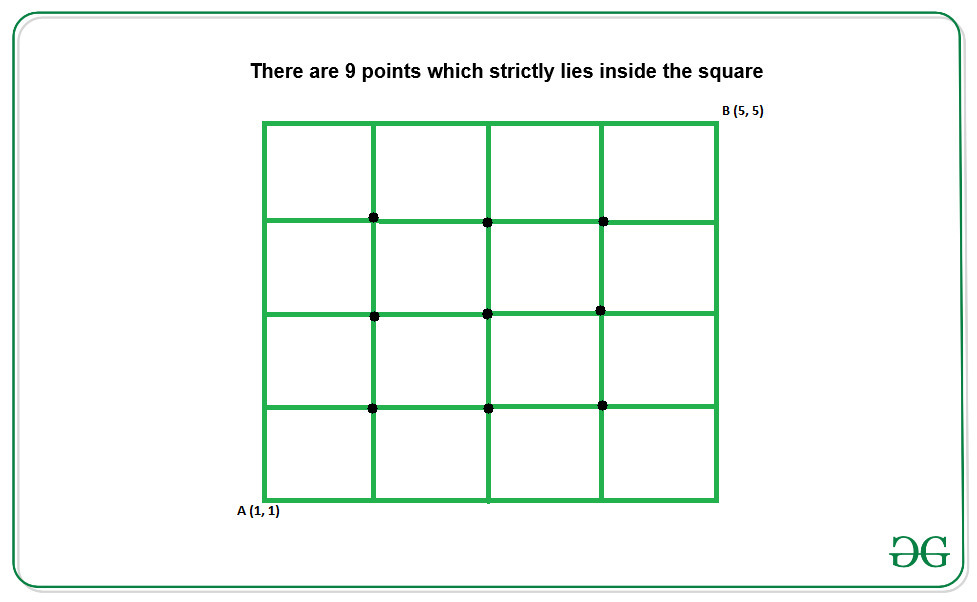# Count of integral coordinates that lies inside a Square

Given lower left and upper right coordinates (x1, y1) and (x2, y2) of a square, the task is to count the number of integral coordinates that lies strictly inside the square.

Examples:

Input: x1 = 1, y1 = 1, x2 = 5, x3 = 5
Output: 9
Explanation:
Below is the square for the given coordinates:Input: x1 = 1, y1 = 1, x2 = 4, x3 = 4
Output: 4

## Recommended: Please try your approach on {IDE} first, before moving on to the solution.

Approach: The difference between the x and y ordinates of the lower and upper right coordinates of the given squares gives the number integral points of x ordinates and y ordinates between opposite sides of square respectively. The total number of points that strictly lies inside the square is given by:

count = (x2 – x1 – 1) * (y2 – y1 – 1)

For Example:In the above figure:
1. The total number of integral points inside base of the square is (x2 – x1 – 1).
2. The total number of integral points inside height of the square is (y2 – y1 – 1).
These (x2 – x1 – 1) integrals points parallel to the base of the square repeats (y2 – y1 – 1) number of times. Therefore the total number of integral points is given by (x2 – x1 – 1)*(y2 – y1 – 1).

Below is the implementation of the above approach:

## C++

 `// C++ program for the above approach ` `#include ` `using` `namespace` `std; ` ` `  `// Function to calculate the integral ` `// points inside a square ` `void` `countIntgralPoints(``int` `x1, ``int` `y1, ` `                        ``int` `x2, ``int` `y2) ` `{ ` `    ``cout << (y2 - y1 - 1) * (x2 - x1 - 1); ` `} ` ` `  `// Driver Code ` `int` `main() ` `{ ` `    ``int` `x1 = 1, y1 = 1; ` `    ``int` `x2 = 4, y2 = 4; ` ` `  `    ``countIntgralPoints(x1, y1, x2, y2); ` `    ``return` `0; ` `} `

## Java

 `// Java program for the above approach ` ` `  `class` `GFG { ` ` `  `// Function to calculate the integral  ` `// points inside a square  ` `static` `void` `countIntgralPoints(``int` `x1, ``int` `y1,  ` `                               ``int` `x2, ``int` `y2) ` `{ ` `    ``System.out.println((y2 - y1 - ``1``) * ` `                       ``(x2 - x1 - ``1``)); ` `} ` ` `  `// Driver Code ` `public` `static` `void` `main(String args[]) ` `{ ` `    ``int` `x1 = ``1``, y1 = ``1``; ` `    ``int` `x2 = ``4``, y2 = ``4``; ` `     `  `    ``countIntgralPoints(x1, y1, x2, y2); ` `} ` `} ` ` `  `// This code is contributed by rutvik_56 `

## Python3

 `# Python3 program for the above approach ` ` `  `# Function to calculate the integral ` `# points inside a square ` `def` `countIntgralPoints(x1, y1, x2, y2): ` `    ``print``((y2 ``-` `y1 ``-` `1``) ``*` `(x2 ``-` `x1 ``-` `1``)) ` ` `  `# Driver Code ` `if` `__name__ ``=``=` `'__main__'``: ` ` `  `    ``x1 ``=` `1` `    ``y1 ``=` `1` `    ``x2 ``=` `4` `    ``y2 ``=` `4` ` `  `    ``countIntgralPoints(x1, y1, x2, y2) ` ` `  `# This code is contributed by Samarth `

## C#

 `// C# program for the above approach ` `using` `System; ` ` `  `class` `GFG{ ` `     `  `// Function to calculate the integral  ` `// points inside a square  ` `static` `void` `countIntgralPoints(``int` `x1, ``int` `y1,  ` `                               ``int` `x2, ``int` `y2)  ` `{  ` `    ``Console.WriteLine((y2 - y1 - 1) *  ` `                      ``(x2 - x1 - 1));  ` `}  ` ` `  `// Driver code ` `static` `void` `Main() ` `{ ` `    ``int` `x1 = 1, y1 = 1;  ` `    ``int` `x2 = 4, y2 = 4;  ` `     `  `    ``countIntgralPoints(x1, y1, x2, y2);  ` `} ` `} ` ` `  `// This code is contributed by divyeshrabadiya07     `

Output:

```4
```

Time Complexity: O(1)

Don’t stop now and take your learning to the next level. Learn all the important concepts of Data Structures and Algorithms with the help of the most trusted course: DSA Self Paced. Become industry ready at a student-friendly price.

My Personal Notes arrow_drop_upCheck out this Author's contributed articles.

If you like GeeksforGeeks and would like to contribute, you can also write an article using contribute.geeksforgeeks.org or mail your article to contribute@geeksforgeeks.org. See your article appearing on the GeeksforGeeks main page and help other Geeks.

Please Improve this article if you find anything incorrect by clicking on the "Improve Article" button below.